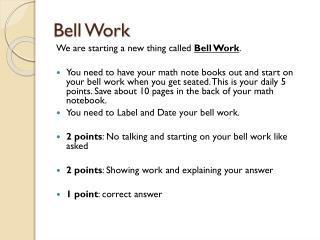# Bell Work - PowerPoint PPT PresentationDownload PresentationBell Work

Bell Work
Download Presentation## Bell Work

- - - - - - - - - - - - - - - - - - - - - - - - - - - E N D - - - - - - - - - - - - - - - - - - - - - - - - - - -
##### Presentation Transcript

2. Bell Work • When given the equation ½ x + 5 = 20, solve for the variable “x” and explain how you solve the equation.

3. Solving Inequalities Math notebook, pencil and protractor

4. Solving Inequalities

5. Goal • Our goal in solving inequalities is the same as solving equations solving for our variable. • Our aim is to have ”x” (or whatever the variable is) on its own on the left of the inequality sign: • Example:  x < 5 or  y ≥ 11

6. Solving Solving inequalities is very like solving inequalities but you must also pay attention to the direction of the inequality. Some things you do will change the direction! < would become > > would become < ≤ would become ≥ ≥ would become ≤

7. Play it safe! These are things you can do without affecting the direction of the inequality: • Add (or subtract) a number from both sides • Multiply (or divide) both sides by a positive number • Simplify a side Example: 3x < 10+5 You can simplify 10 and 5 without affecting the inequality: 3x < 15

8. When you can’t play it safe… But these things will change the direction of the inequality ("<" becomes ">" for example): • Multiply (or divide) both sides by a negative number • Swapping left and right hand sides Example: 2y+7 < 14 When you swap the left and right hand sides, you must also change the direction of the inequality: 14> 2y+7

9. Adding & Subtracting • We can often solve inequalities by adding (or subtracting) a number from both sides • Example: x – 3 < 8 If you add (or subtract) the same amount from both sides, it does not affect the inequality

10. Example • Alex has more coins than Billy. If both Alex and Billy get three more coins each, Alex will still have more coins than Billy. • Pick a variable for each boy. (a = amount for Alex, b = amount for Billy)

11. Alex has more coins than Billy. Write an inequality; a > b If both Alex and Billy get three more coins each Add to your inequality: A + 3 > b +3

12. What If I Solve It, But "x" Is On The Right? • Just swap sides, but reverse the sign so it still "points at" the correct value! • Example: 12 < x + 5 • If we subtract 5 from both sides, we get: 12 - 5 < x + 5 - 5  = 7 < x

13. But it is normal to put "x" on the left hand side...so let us flip sides (and the inequality sign!): x > 7 • Do you see how the inequality sign still "points at" the smaller value (7) ? • And that is our solution:  x > 7 Note: "x" can be on the right, but people usually like to see it on the left hand side.

14. Open vs Closed Circles on Number Line • If your symbol has the "equal to option," which is that single line underneath the greater than/less than symbol, then you use a closed circle.   • The closed circle is only used for the  or  symbols.   The open circle is used for >, < and

15. Example:   x > -2 We read this inequality as x is "greater than" negative 2.  (The mouth is facing the x). Since our inequality does NOT have the equal to option, we use an open circle. Since our inequality reads as "greater than," we shade to the right.

16. Examples 1. 4  > x (Graph on a number line) • X + 3 < 10 (Graph on a number line) • X – 4 ≥ 12 (Graph on a number line)